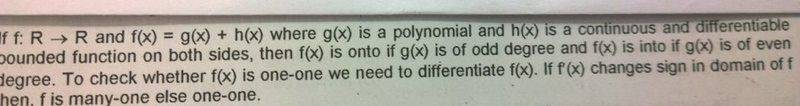# Checking if f(x)=g(x)+h(x) is onto

• Titan97
Gold Member
This is picture taken from my textbook.I understood the last two statements "To check whether..". A function is one if its strictly increasing or decreasing. But I am not able to understand the first statement. Polynomials are continuous functions. Also, a continuous function ± discontinuous function may be continuous. (eg: {x}+[x]) So why should h(x) necessarily be a continuous function?

Mentor
It does not make any statement for discontinuous functions. Sure, there are discontinuous h(x) that would still work, but not in the general case.

Unrelated:
The sum of a continuous function and a discontinuous function is discontinuous. Your example sums two discontinuous functions.

•Titan97
Gold Member
So h(x) has to be continuous. (I got cinfused while typing). But what makes f(x) onto?

Mentor
What do you know if g(x) is a polynomial of odd degree?
Does the information about continuity and bounds change if you add a bound continuous function?

Gold Member
For polynomials of odd degree, the range is (-∞,∞). If h(x) is only defined ∀ x∈[a,b], then f(x)=g(x)+h(x) is only defined ∀ x∈(a,b).

Mentor
That's not how "bounded function" is meant here. Its function values are limited, not its domain.

•Titan97
Gold Member
The continuity won't change if you add such a function like x+sinx

Staff Emeritus
Homework Helper
Given a polynomial of odd degree ##P(x)##. Think about
$$\lim_{x\rightarrow +\infty} P(x)~\text{and}~\lim_{x\rightarrow -\infty} P(x)$$
Can those be equal? Can you give an example when those limits will be equal?

•Titan97
Gold Member
They won't be equal

Staff Emeritus
Homework Helper
So what will they be concretely? Can you deduce from that that the function ##P(x)## is onto?

•Titan97
Gold Member
Yes.

Staff Emeritus
•